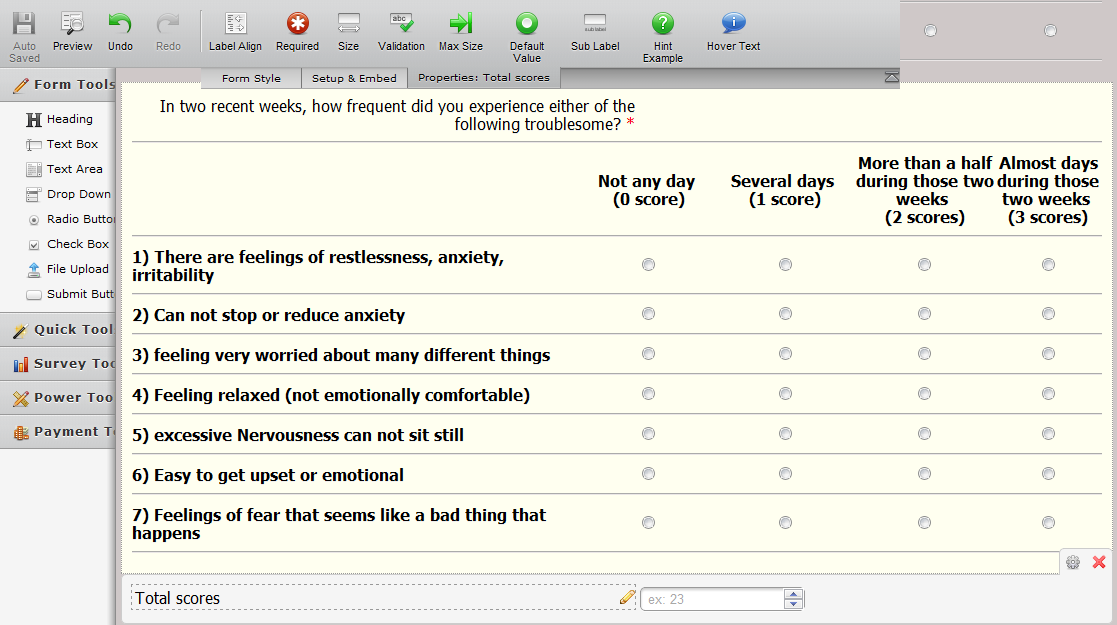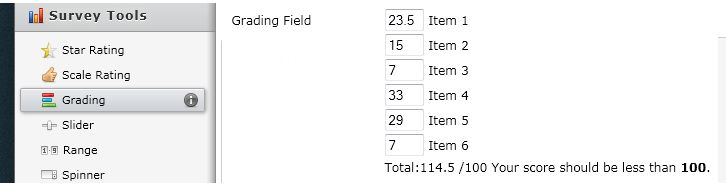# Can we insert summary function to calculate scores for matrix form?

•theanhdbt
Asked on March 19, 2012 at 11:35 PM

Can I create a Matrix table which has summary function for each column?

Let say I've got five rows named row 1, row 2, row 3, row 4, row 5 and three columns named Col 1, Col 2, Col 3 in which:

+ five rows provide me five questions to be answer by respondent and

+ three columns present for three options for each answer,

and each option was assigned a score (let say Col 1: score=0, Col 2: score=1, Col 3: score=2)

All things I want is making the score to appear automatically right after the respondent filling in all rows' answer (select answer in either of the columns) by summing all scores in the matrix (in order to help the handling person with this form immediately give his decision based on the total score)

Thanks so much for any info about how to do that in jotform

Wish the website become more popular and developed.

Best Regards,

The Anh•gori-mathew
Answered on March 20, 2012 at 01:30 AM

Hello,

Thanks for the much compliments you have about our platform, we feel gald to know how much Jotform is becoming the choice for many users.

With the current version of Jotform, we only have two known types of fields that can calculate the sum of inputted values. These are payment field and grading field.Unfortunately, we do not have a feature that calculates total in other fields such as the matrix field.

Please let us know if you have further questions. Thank you for using Jotform!

Mathew